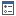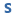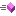﻿ LinearUnit Class Members—ArcGIS Pro
ArcGIS Pro 2.6 API Reference Guide
LinearUnit Class Members
Properties  Methods

 ArcGIS.Core.Geometry Namespace : LinearUnit Class

The following tables list the members exposed by LinearUnit.

Public Properties
NameDescriptionGets a linear unit representing centimeters (Factory code = 109006).Gets the conversion factor of the unit. (Inherited from ArcGIS.Core.Geometry.Unit)Gets a linear unit representing decimeters (Factory code = 109005).Gets the well-known ID of the unit. If the unit is a custom unit, then the factory code will be 0. (Inherited from ArcGIS.Core.Geometry.Unit)Gets a linear unit representing feet (Factory code = 9002).Gets a linear unit representing inches (Factory code = 109008).Gets a linear unit representing kilometers (Factory code = 9036).Gets a linear unit representing meters (Factory code = 9001).Gets the meters per unit.Gets a linear unit representing miles (Factory code = 9093).Gets a linear unit representing millimeters (Factory code = 109007).Gets the name of the unit. (Inherited from ArcGIS.Core.Geometry.Unit)Gets a linear unit representing international nautical miles (Factory code = 9030).Gets a linear unit representing points (Factory code = 109016).Gets the type of unit. (Inherited from ArcGIS.Core.Geometry.Unit)Gets the well-known text of the unit. (Inherited from ArcGIS.Core.Geometry.Unit)Gets a linear unit representing yards (Factory code = 109001).
Top
Public Methods
NameDescriptionConverts a value in meters to the unit of this instance.Converts a value in the unit of this instance to the provided linear unit.Converts a value in the unit of this instance to meters.Overloaded.Gets the JSON representation of the unit in terms of the wkid. A custom unit has wkid = 0. (Inherited from ArcGIS.Core.Geometry.Unit)Gets a System.String that represents this unit. Returns the Unit.Name. (Inherited from ArcGIS.Core.Geometry.Unit)
Top